# Quiz 13: Finding Differences

Nature of the scale: Scale is used to perform the differences test between the companies. The scales are of two types: (i) categorical scale and (ii) metric scale. Categorical scale: The researcher compares two or more companies based on the percentage called categorical scale. Consider the formula for percentage-based comparison.Where, P 1 is percent for sample 1 and q 1 = 100 - P 1. P 2 is percent for sample 2 and q 2 = 100 - P 2. Metric scale: The researcher compares two or more companies based on average of companies called Metric scale. Consider the formula for average-based comparison.Where,is denoted as average of sample 1.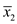is denoted as average of sample 2.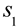is denoted as the standard deviation of sample 1.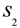is denoted as the standard deviation of sample 2.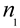is denoted as size of sample 1.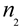is denoted as size of sample 2. Thus, the two scales used to find the difference between the companies was discussed.